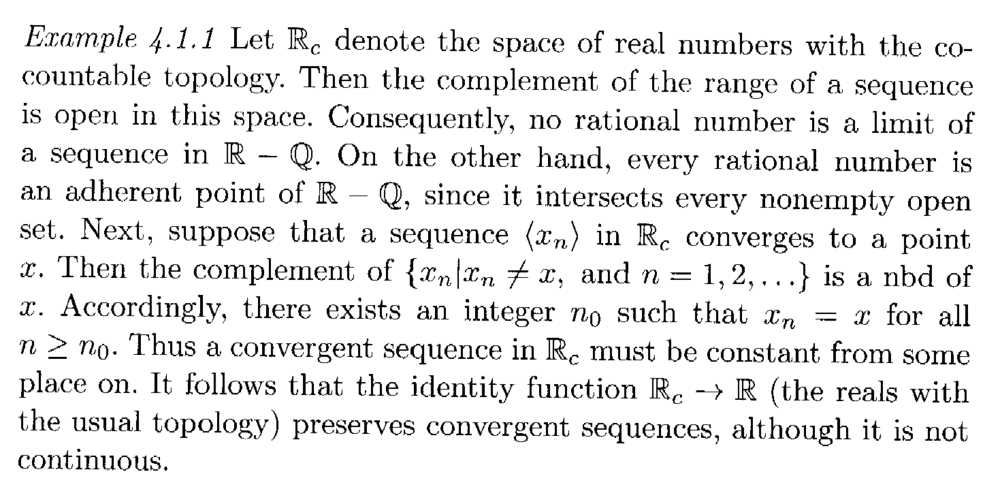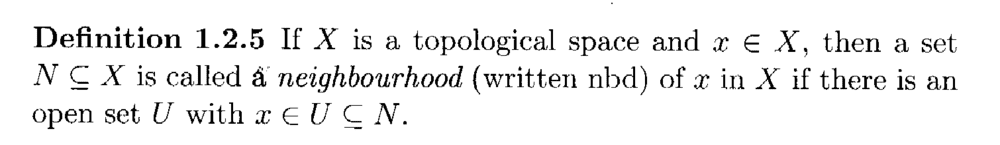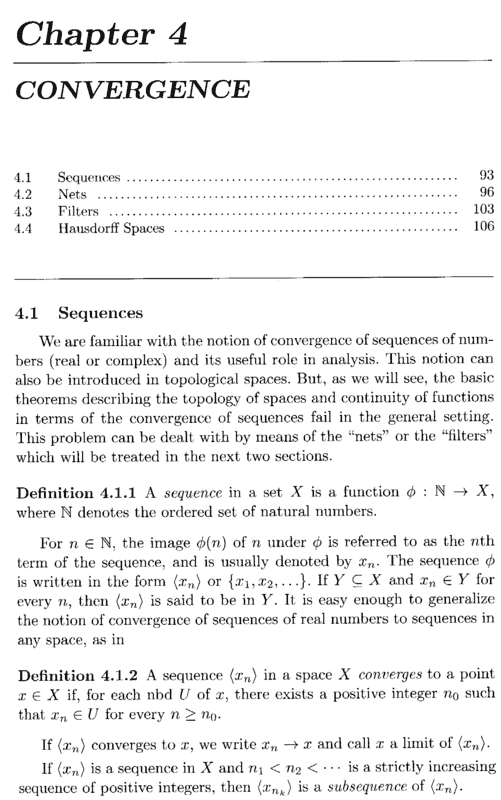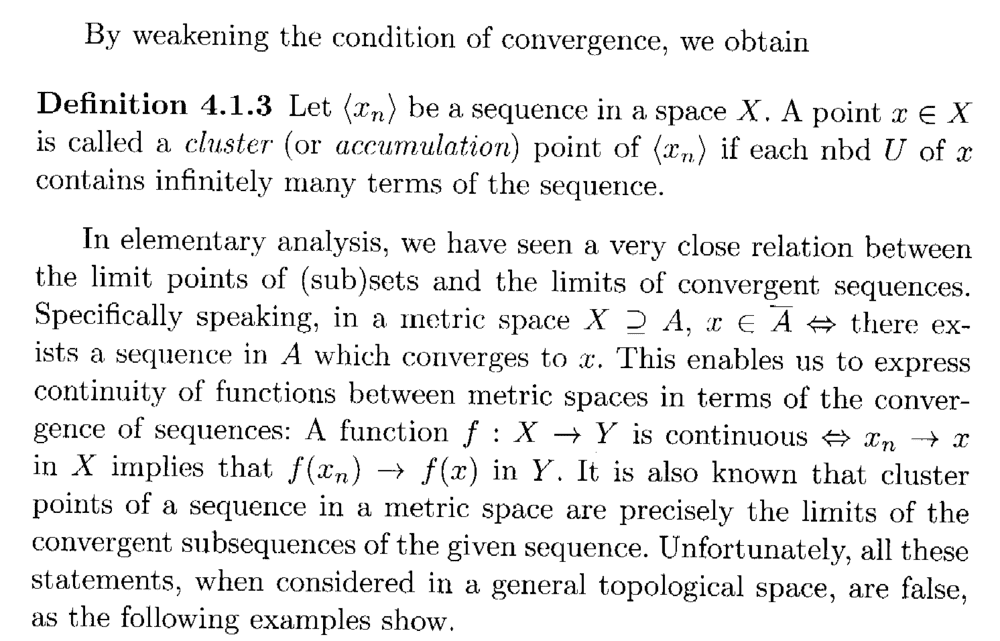# Convergence .... Singh, Example 4.1.1 .... .... Another Question ....

• I
• Math Amateur

#### Math Amateur

Gold Member
MHB
TL;DR Summary
I need further help in order to fully understand an example concerning convergence in the space of real numbers with the co-countable topology ...
I am reading Tej Bahadur Singh: Elements of Topology, CRC Press, 2013 ... ... and am currently focused on Chapter 4, Section 4.1: Sequences ...

I need some further help in order to fully understand Example 4.1.1 ...In the above example from Singh we read the following:

" ... ...Then the complement of ##\{ x_n \ | \ x_n \neq x \text{ and } n = 1,2, ... \}## is a nbd of ##x##. Accordingly, there exists an integer ##n_0## such that ##x_n = x## for all ##n \geq n_0##. ... ... "

My question is as follows:

Why, if the complement of ##\{ x_n \ | \ x_n \neq x## and ##n = 1,2, ... \}## is a nbd of ##x## does there exist an integer ##n_0## such that ##x_n = x## for all ##n \geq n_0##. ... ... ?

Help will be much appreciated ... ...

Peter

=====================================================================================

It may help readers of the above post to have access to Singh's definition of a neighborhood and to the start of Chapter 4 (which gives the relevant definitions) ... so I am providing the text as follows:Hope that helps ...

Peter

##V=\mathbb{R} \setminus \{x_n: x \neq x_n, n \geq 1\}## is a neighborhood of ##x## as ##V## contains ##x## and it is the complement of a countable set, thus open.

But ##x_n \to x##, which means that for all neighborhoods ##U## of ##X##, there is ##n_0## such that ##x_n \in U## if ##n \geq n_0##.

Next, take ##U = V##. Then there is ##n_0## such that ##x_n \in V \iff x_n = x## if ##n \geq n_0##.

__________________

Note that the converse of what the author claims holds: any sequence that is eventually constant converges (to the eventually constant value). Thus in this topological space, the convergent sequences are exactly these which are eventually constant.

On ##\mathbb{R}##, we can also define the discrete topology ##\mathcal{P}(\mathbb{R})## (every subset of ##\mathbb{R}## is open). Then also a sequence in ##\mathbb{R}## is convergent if and only if it is eventually constant (easy exercise).

Thus we see that ##(\mathbb{R}, \mathcal{T}_c)## and ##(\mathbb{R}, \mathcal{P}(\mathbb{R}))## are different topological spaces with the same convergent sequences.

This means that sequences do not suffice to describe the topology (unlike for metric spaces or more generally spaces where every point has a countable neighborhood basis). The solution then is to generalise the concept of sequence and allow more general index sets than ##\mathbb{N}##. We then come to the concept of nets and these do describe the topology. But some authors use other approaches and use filters instead of net, though both concepts are equivalent and can be translated to one another.

TLDR: Sequences in topological spaces do not suffice to describe the topology, so there is the need to introduce a new kind of object to describe convergence. This will be a net or a filter.

Last edited by a moderator:
•Math Amateur
Thanks for the help, Math_QED ...

And thanks also for a most interesting post ...

Peter

•member 587159